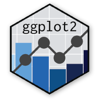Show Sidebar Hide Sidebar# axis.title in ggplot2

How to modify axis titles in R and ggplot2.

### New to Plotly?

Plotly's R library is free and open source!
You can set up Plotly to work in online or offline mode.
We also have a quick-reference cheatsheet (new!) to help you get started!

### Version Check

Version 4 of Plotly's R package is now available!
Check out this post for more information on breaking changes and new features available in this version.

library(plotly)
packageVersion('plotly')

##  '4.9.1'


### Axis Labels

library(plotly)
set.seed(123)

df <- diamonds[sample(1:nrow(diamonds), size = 1000),]

# To set x-axis and y-axis labels use labs()
p <- ggplot(df, aes(carat, price)) +
geom_point() +
labs(title = "Diamonds", x = "x-axis -> Carat", y = "y-axis -> Price")

p <- ggplotly(p)

# Alternatively use
p <- ggplot(df, aes(carat, price)) +
geom_point() +
ggtitle("Diamonds") +
xlab("x-axis -> Carat") +
ylab("y-axis -> Price")

p <- ggplotly(p)

p


### Axis Title Size

library(plotly)
set.seed(123)

df <- diamonds[sample(1:nrow(diamonds), size = 1000),]

p <- ggplot(df, aes(carat, price)) +
geom_point() +
labs(title = "Diamonds", x = "x-axis -> Carat", y = "y-axis -> Price") +
theme(plot.title = element_text(size = 50),
axis.title.x = element_text(size = 20),
axis.title.y = element_text(size = 20))

p <- ggplotly(p)

p


### Axis Title Colors

library(plotly)
set.seed(123)

df <- diamonds[sample(1:nrow(diamonds), size = 1000),]

p <- ggplot(df, aes(carat, price)) +
geom_point() +
labs(title = "Diamonds", x = "x-axis -> Carat", y = "y-axis -> Price") +
theme(plot.title = element_text(size = 50, colour = "#668cff"),
axis.title.x = element_text(size = 20, colour = "#6699ff"),
axis.title.y = element_text(size = 20, colour = "#ff8080"))

p <- ggplotly(p)

p


Inspired by ggplot2 documentation